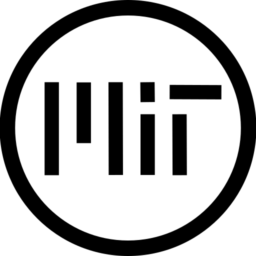# Depth Modulated Pixel Outline in Screen Space

This shader uses a  linearize depth texture, which is essential when writing depth shaders in godot 4.x, as the new implementation using vulcan changes how the depth buffer works since 3.x.

It also uses the cull_front render mode and it automatically ‘flips face’ of the screen space quad, it has not been documented yet that this is necesarry in godot 4.0.

It’s main thing is that it increases the intensity of the edges if the neighboring fragments are further away. Which gives objects a bit more contrast.

Params are
– Outline Color
– Distance Falloff
– Smoothing Cutoff
– Smoothing Max

It works well in both perspective and orthographic camera modes.

This is a screen space spacial shader which uses depth, and that means this shader requires the use of the the screen-space quad workaround documented here.

Additionally to this documentation, you may need to rotate the quad 180 degrees around the Y for the fullscreen mode (toggled via comment in the vertex shader) to be consistent with non-fullscreen mode.

``````shader_type spatial;

uniform vec4 outline_color : source_color = vec4(0.0, 0.0, 0.0, 1.0);
uniform float distance_falloff : hint_range(0, 5) = 1;
uniform float smoothing_cutoff : hint_range(0, 1) = 0.1;
uniform float smoothing_max : hint_range(0, 1) = 0.1;

void vertex() {
// remember to rotate the quad 180d around Y

// uncomment to use full screen
//POSITION = vec4(VERTEX, 1.0) * vec4(-1.0, -1.0, 1.0, 1.0);
}

float abs_diff(float depth_a, float depth_b){
return abs(abs(depth_a)-abs(depth_b));
}

float linear_depth(in sampler2D depth_texture, in vec2 screen_uv, in mat4 inv_projection_matrix){
// get raw depth, this is not a linear value in godot 4.0 vulkan rendering
float raw_depth = texture(depth_texture, screen_uv);

vec3 normalized_device_coordinates = vec3(screen_uv * 2.0 - 1.0, raw_depth);

//convert NDC to view space via the inverse projection matrix
vec4 view_space = inv_projection_matrix * vec4(normalized_device_coordinates, 1.0);

//linearize the depth
view_space.xyz /= view_space.w;

// camera view points in the negative Z direction, so all depths are negative
// we invert the sign here to get positive depth values
return -view_space.z;
}

void fragment() {
// set color to outline color for whole screen
// non-outline fragments will be set to transparent
ALBEDO = outline_color.rgb;

float d = linear_depth(DEPTH_TEXTURE, SCREEN_UV, INV_PROJECTION_MATRIX);

// calculate the offset size of a single pixel
vec2 screen_size = vec2(textureSize(SCREEN_TEXTURE, 1));

vec2 pixel_size = (distance_falloff / d) / vec2(screen_size.x, screen_size.y);

float du = linear_depth(DEPTH_TEXTURE, SCREEN_UV+vec2(0.0, pixel_size.y), INV_PROJECTION_MATRIX);
float dd = linear_depth(DEPTH_TEXTURE, SCREEN_UV+vec2(0.0, -pixel_size.y), INV_PROJECTION_MATRIX);
float dr = linear_depth(DEPTH_TEXTURE, SCREEN_UV+vec2(pixel_size.x, 0.0), INV_PROJECTION_MATRIX);
float dl = linear_depth(DEPTH_TEXTURE, SCREEN_UV+vec2(-pixel_size.x, 0.0), INV_PROJECTION_MATRIX);

// combine all the abs differences in depth of neighbors
ALPHA =
abs_diff(d, du) +
abs_diff(d, dl) +
abs_diff(d, dd) +
abs_diff(d, dr)
;

ALPHA = smoothstep(smoothing_cutoff, max(smoothing_cutoff, smoothing_max), ALPHA);

// apply the alpha from the outline color as well
ALPHA *= outline_color.a;

// clamp the outline color to remove artifacts
ALPHA = clamp(ALPHA, 0.0, 1.0);
}``````
###### Tags
depth, edge, edge detection, outline, pixel, screen-spaceThe shader code and all code snippets in this post are under MIT license and can be used freely. Images and videos, and assets depicted in those, do not fall under this license. For more info, see our License terms.

### Grass with Screen-Space DisplacementInline FeedbacksJohn
4 months ago

Are you able to provide an example project? I can’t seem to get this working for the life of me.

Last edited 4 months ago by Johncosmoddd
2 months ago

Doesn’t work. Error message:

SHADER ERROR: DEPTH_TEXTURE has been removed in favor of using hint_depth_texture with a uniform.
To continue with minimal code changes add ‘uniform sampler2D DEPTH_TEXTURE : hint_depth_texture, filter_linear_mipmap;’ near the top of your shader.QuibblingComet
2 months ago

do what the debugger says should fix the issue. Screen texture was replaced with a hintcosmoddd
1 month ago